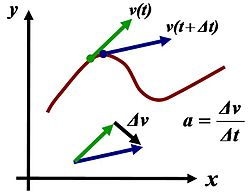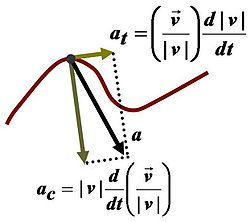# Acceleration

Acceleration

In physics, acceleration is the rate of change of velocity with time. In one dimension, acceleration is the rate at which something speeds up or slows down. However, since velocity is a vector, acceleration describes the rate of change of both the magnitude and the direction of velocity. Acceleration has the dimensions L T −2. In SI units, acceleration is measured in meters per second squared (m/s2). (Negative acceleration i.e. retardation, also has the same dimensions/units.)

Proper acceleration, the acceleration of a body relative to a free-fall condition, is measured by an instrument called an accelerometer.

In common speech, the term acceleration is used for an increase in speed (the magnitude of velocity); a decrease in speed is called deceleration. In physics, a change in the direction of velocity also is an acceleration: for rotary motion, the change in direction of velocity results in centripetal (toward the center) acceleration; whereas the rate of change of speed is a tangential acceleration.

In classical mechanics, for a body with constant mass, the acceleration of the body is proportional to the net force acting on it (Newton's second law):$\mathbf{F} = m\mathbf{a} \quad \to \quad \mathbf{a} = \mathbf{F}/m$

where F is the resultant force acting on the body, m is the mass of the body, and a is its acceleration.Acceleration is the rate of change of velocity. At any point on a trajectory, the magnitude of the acceleration is given by the rate of change of velocity in both magnitude and direction at that point. The true acceleration at time t is found in the limit as time interval Δt → 0.Components of acceleration for a planar curved motion. The tangential component at is due to the change in speed of traversal, and points along the curve in the direction of the velocity vector. The centripetal component ac is due to the change in direction of the velocity vector and is normal to the trajectory, pointing toward the center of curvature of the path.

Average acceleration is the change in velocity (Δv) divided by the change in time (Δt). Instantaneous acceleration is the acceleration at a specific point in time which is for a very short interval of time as Δt approaches zero.

## Tangential and centripetal acceleration

The velocity of a particle moving on a curved path as a function of time can be written as:$\mathbf{v} (t) =v(t) \frac {\mathbf{v}(t)}{v(t)} = v(t) \mathbf{u}_\mathrm{t}(t) ,$

with v(t) equal to the speed of travel along the path, and$\mathbf{u}_\mathrm{t} = \frac {\mathbf{v}(t)}{v(t)} \ ,$

a unit vector tangent to the path pointing in the direction of motion at the chosen moment in time. Taking into account both the changing speed v(t) and the changing direction of ut, the acceleration of a particle moving on a curved path on a planar surface can be written using the chain rule of differentiation and the derivative of the product of two functions of time as:\begin{alignat}{3} \mathbf{a} & = \frac{d \mathbf{v}}{dt} \\ & = \frac{\mathrm{d}v }{\mathrm{d}t} \mathbf{u}_\mathrm{t} +v(t)\frac{d \mathbf{u}_\mathrm{t}}{dt} \\ & = \frac{\mathrm{d}v }{\mathrm{d}t} \mathbf{u}_\mathrm{t}+ \frac{v^2}{R}\mathbf{u}_\mathrm{n}\ , \\ \end{alignat}

where un is the unit (inward) normal vector to the particle's trajectory, and R is its instantaneous radius of curvature based upon the osculating circle at time t. These components are called the tangential acceleration and the radial acceleration or centripetal acceleration (see also circular motion and centripetal force).

Extension of this approach to three-dimensional space curves that cannot be contained on a planar surface leads to the Frenet–Serret formulas.

## Special cases

### Uniform acceleration

Uniform or constant acceleration is a type of motion in which the velocity of an object changes by an equal amount in every equal time period.

A frequently cited example of uniform acceleration is that of an object in free fall in a uniform gravitational field. The acceleration of a falling body in the absence of resistances to motion is dependent only on the gravitational field strength g (also called acceleration due to gravity). By Newton's Second Law the force, F, acting on a body is given by:$\mathbf {F} = m \mathbf {g}$

Due to the simple algebraic properties of constant acceleration in the one-dimensional case (that is, the case of acceleration aligned with the initial velocity), there are simple formulas that relate the following quantities: displacement, initial velocity, final velocity, acceleration, and time:$\mathbf {v}= \mathbf {u} + \mathbf {a} t$$\mathbf {s}= \mathbf {u} t+ {{1} \over {2}} \mathbf {a}t^2 = {{(\mathbf{u}+\mathbf{v})t} \over {2}}$$|\mathbf {v}|^2= |\mathbf {u}|^2 + 2 \, \mathbf {a} \cdot \mathbf {s}$

where$\mathbf{s}$ = displacement$\mathbf{u}$ = initial velocity$\mathbf{v}$ = final velocity$\mathbf{a}$ = uniform acceleration
t = time.

In the case of uniform acceleration of an object that is initially moving in a direction not aligned with the acceleration, the motion can be resolved into two orthogonal parts, one of constant velocity and the other according to the above equations. As Galileo showed, the net result is parabolic motion, as in the trajectory of a cannonball, neglecting air resistance.

### Circular motion

An example of a body experiencing acceleration of a uniform magnitude but changing direction is uniform circular motion. In this case, because the direction of the object's motion is constantly changing, being tangential to the circle, the object's velocity also changes, but its speed does not. This acceleration is directed toward the centre of the circle and takes the value:$a = {{v^2} \over {r}}$

where v is the object's speed. Equivalently, the radial acceleration may be calculated from the object's angular velocity ω, whence:$\mathbf {a}= {-\omega^2} \mathbf {r}.$

The acceleration, hence also the force acting on a body in uniform circular motion, is directed toward the center of the circle; that is, it is centripetal – the so called 'centrifugal force' appearing to act outward on a body is really a pseudo force experienced in the frame of reference of the body in circular motion, due to the body's linear momentum at a tangent to the circle.

## Relation to relativity

After completing his theory of special relativity, Albert Einstein realized that forces felt by objects undergoing constant proper acceleration are actually feeling themselves being accelerated, so that, for example, a car's forward acceleration would result in the driver feeling a slight pressure between himself and his seat. In the case of gravity, which Einstein concluded is not actually a force, this is not the case; acceleration due to gravity is not felt by an object in free-fall. The reason for this difference is that in the case of the car the force due to the engine is applied directly only to a certain part of the mass while the driver and the bulk of the car are passively dragged along. Gravity on the other hand accelerates the entire mass, with no internal forces acting. This was the basis for his development of general relativity, a relativistic theory of gravity.

Wikimedia Foundation. 2010.

Synonyms:

### Look at other dictionaries:

• accélération — [ akselerasjɔ̃ ] n. f. • 1327; lat. acceleratio, de accelerare 1 ♦ Cour. Augmentation de vitesse. L accélération d un mouvement. Cette voiture a des accélérations foudroyantes. Fig. Le fait d aller plus vite. L accélération du pouls, de la… …   Encyclopédie Universelle

• Acceleration — Accélération L accélération désigne couramment une augmentation de la vitesse; en physique, plus précisément en cinématique, l accélération est une grandeur vectorielle qui indique la modification affectant la vitesse d un mouvement en fonction… …   Wikipédia en Français

• acceleration — I noun dispatch, expedition, expeditious performance, hastening, hurrying, increase of speed, quickening, shortening of time, speedup, spurt, stepping up a pace associated concepts: acceleration clause, acceleration doctrine, acceleration of a… …   Law dictionary

• Acceleration — Ac*cel er*a tion, n. [L. acceleratio: cf. F. acc[ e]l[ e]ration.] The act of accelerating, or the state of being accelerated; increase of motion or action; as, a falling body moves toward the earth with an acceleration of velocity; opposed to… …   The Collaborative International Dictionary of English

• Acceleration — Ac*cel er*a tion, n. [L. acceleratio: cf. F. acc[ e]l[ e]ration.] The act of accelerating, or the state of being accelerated; increase of motion or action; as, a falling body moves toward the earth with an acceleration of velocity; opposed to… …   The Collaborative International Dictionary of English

• acceleration — of a point is the time rate of change of the velocity of the point. centripetal acceleration lateral acceleration longitudinal acceleration normal acceleration side acceleration vehicle acceleration …   Mechanics glossary

• acceleration — The termination of the debtor s right to pay a debt in installments or at a future maturity date. If a debt is accelerated, the debt becomes immediately due and payable. Many contracts have acceleration clauses entitling the creditor to allow for …   Glossary of Bankruptcy

• Acceleration — Acceleration, Beschleunigung, beschleunigende Kraft, die ein Körper auf die Bewegung eines andern ausübt. – 2. Acceleration des Mondes nennt die Astronomie die Beschleunigung des Mondlaufes um die Erde, in Folge deren dieser immer kürzer wird,… …   Herders Conversations-Lexikon

• acceleration — Acceleration. subst. f. v. L action d accelerer. Il faut faire telle chose pour l acceleration de l ouvrage …   Dictionnaire de l'Académie française

• Acceleration — (v. lat.), 1) Beschleunigung; bes. 2) Vermehrung der Geschwindigkeit; so: A. des Mondes, die immer größer werdende Geschwindigkeit des Mondes, od. die immer kürzere Umlaufszeit des Mondes um die Erde, s. u. Mond; A. der Fixsterne, Verfrühung der… …   Pierer's Universal-Lexikon

• Acceleration — Acceleration, s. Beschleunigung …   Lexikon der gesamten Technik